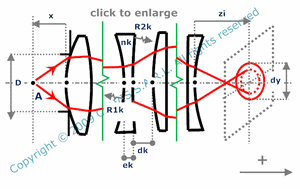and orgeometrical optics
spherical aberration

light spot induced by a combination of plane parallel plates and spherical lenses for an object on the axis

 number of lensesR1k : radius of curvature of the first surface of lens number k R2k : radius of curvature of the second surface of lens number k ek : center thickness of lens number k nk : refraction index of lens number k dk : distance from the second surface of lens number k to the first surface of lens number k+1 x : distance from the vertex of the first surface to the object point D : diameter of the aperture located on the first surface ( located on the first surface and not in the plane tangent to the first surface and passing through the apex ) zi : distance from the vertex of the last surface to the considered plane in the image space dy : spot diameter in the considered plane

Note
This calculation page can not detect all unrealistic inputs.